﻿ Math8 Assignments
 FAIL (the browser should render some flash content, not this).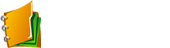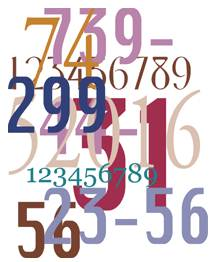Math 8 Ch 1: NumbEr Sense Multiplying and Dividing Integers

Assignments

•  Unit 1.1b Positive & Negative Numbers
•  Unit 1.2 Multiplying Integers with Area Model
•  Unit 1.3 Multiplying Two Digit Integers
•  Unit 1.3b Strategies for Multiplying

Practice Test and Quiz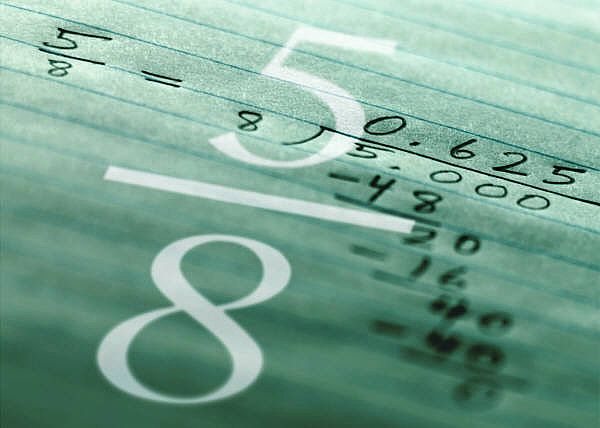Math 8 Ch 2: Fractions and BEDMAS

Assignments

Practice Test and QuizMath 8 Ch 3: Percentages

Assignments

Practice Test and QuizMath 8 Ch 4: Rates and Ratios

Assignments

Practice Test and Quiz

•  Chapter 4 ReviewMath 8 Ch 5: Reading Graphs and Interpreting Data

Assignments

•  5.3 Analyzing Graphs and Data
•  5.4 Mean, Median, and Mode

SOL

5.1
5.2
5.3
5.4

Practice Test and QuizMath 8 Ch 6: Probability

Assignments

SOL

6.1
6.2
6.3
6.4

Practice Test and Quiz

•  Probability Quiz
•  Quiz 6.2 to 6.3
•  Ch 6 Practice Test A
•  Ch 6 Practice Test B
•  Challenging Questions on Probability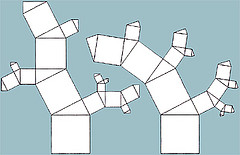Math 8 Ch 7: Pythagorean Properties

Assignments

Practice Test and QuizMath 8 Ch 8: Surface Area of 3D Solids

Assignments

Practice Test and Quiz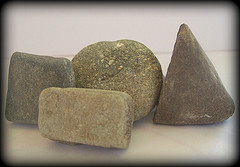Math 8 Ch 9: Volume of 3D Solids

Assignments

Practice Test and QuizMath 8 Ch 10: Linear Relations

Assignments

SOL

10.1
10.2
10.3

Practice Test and Quiz

•  Online Quiz Table of Values
•  Quiz 10.3 to 10.4
•  Ch 10 Practice Test A
•  Video: How to Complete a Table of Values
•  Written Notes from Class
•  Online Lessons
•  Ch 10 Review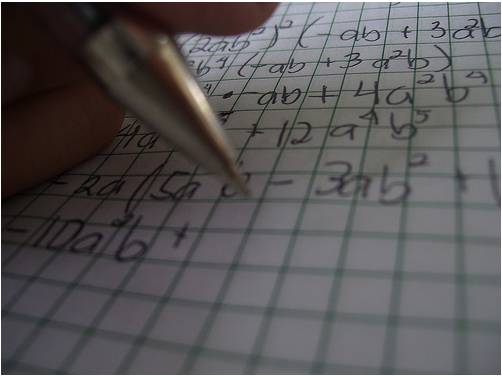Math 8 Ch 11: Solving Linear Relations

Assignments

Practice Quizzes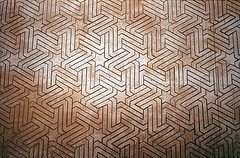Math 8 Ch 12: Tesselation

Assignments

•  12.1 Exploring Tesselations
•  12.2 Constructing Tesselations
•  12.3 Constructing Tesselations (Part 2)

SOL

12.1
12.2
12.3

Practice Test and Quiz

•  Quiz 12.1 to 12.2
•  Quiz 12.3 to 12.4
•  Ch 12 Practice Test A
•  Ch 12 Practice Test B
•  Ch 12 Reviewe